Courses

# Structure Of Atom MCQ - 1 (Advance)

## 25 Questions MCQ Test Chemistry for JEE Advanced | Structure Of Atom MCQ - 1 (Advance)

Description
This mock test of Structure Of Atom MCQ - 1 (Advance) for JEE helps you for every JEE entrance exam. This contains 25 Multiple Choice Questions for JEE Structure Of Atom MCQ - 1 (Advance) (mcq) to study with solutions a complete question bank. The solved questions answers in this Structure Of Atom MCQ - 1 (Advance) quiz give you a good mix of easy questions and tough questions. JEE students definitely take this Structure Of Atom MCQ - 1 (Advance) exercise for a better result in the exam. You can find other Structure Of Atom MCQ - 1 (Advance) extra questions, long questions & short questions for JEE on EduRev as well by searching above.
*Multiple options can be correct
QUESTION: 1

### When photons of energy 4.25 eV strike the surface of a metal A, the ejected photoelectrons have maximum kinetic energy TA eV and de Broglie wavelength λA. The maximum kinetic energy of photoelectrons liberated from another metal B by photons of energy 4.70 eV is TB = (TA - 1.50) eV. If the de Broglie wavelength of these photoelectrons is λB = 2λA, then select the correct statement (s)

Solution: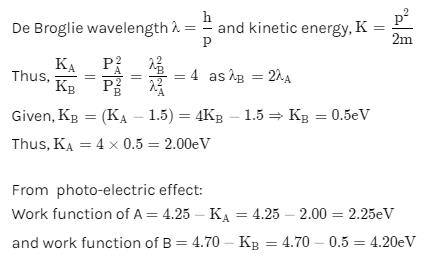*Multiple options can be correct
QUESTION: 2

### Choose the correct statement among the following :

Solution:
*Multiple options can be correct
QUESTION: 3

### Correct statement(s) regarding 3py orbital is/are

Solution: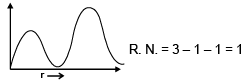Peaks = 1 + 1 =  2

*Multiple options can be correct
QUESTION: 4

Select the correct statement(s) :

Solution:

Electromagnetic waves include visible light, radio waves, X-rays, and so on. What distinguishes these different bands of light is their frequency (or wavelength). But what they all have in common is that they travel at the same speed in vacuum.
Both Heand H , contains 1 electron. So, their spectrum will be similar.

*Multiple options can be correct
QUESTION: 5

Choose the incorrect statement(s) :

Solution:
*Multiple options can be correct
QUESTION: 6

Select the correct curve(s) :

If v = velocity of electron in Bohr's orbit.

r = Radius of electron in Bohr's orbit.

P.E. = Potential energy of electron in Bohr's orbit.

K.E. = Kinetic energy of electron in Bohr's orbit.

Solution: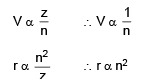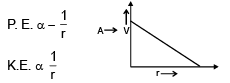*Multiple options can be correct
QUESTION: 7

Which is/are correct statement.

Solution: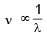QUESTION: 8

It is a data sufficiency problem in which it is to be decided on the basis of given statements whether the given question can be answered or not. No matter whether the answer is yes or no.

Question : Is the orbital of hydrogen atom 3px?

Statement - 1 : The radial function of the orbital is R(r) =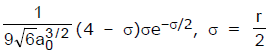Statement - 2 : The orbital has 1 radial node & 0 angular node.

Solution:
QUESTION: 9

Statement - 1 : Energy emitted when an electron jump from 5 → 2 (energy level) is less than when an electron jump from 2 → 1 in all `H' like atom.

Statement - 2 : The |total energy difference| between 1st & 2nd energy level is greater than that of any two energy level provided level `1' is not part of those two energy levels

Solution:
QUESTION: 10

Statement - 1 : Emitted radiations will fall in visible range when an electron jump from higher level to n = 2 in Li+2 ion.

Statement - 2 : Balmer series radiations belong to visible range in all H - like atoms.

Solution:
QUESTION: 11

Assertion : The fraction of total number of electrons in p-sub levels of Mg is 50%

Reason : The fraction of total number of electron in p-sub levels of Be is 40%

Solution:
QUESTION: 12

Assertion : Energy of an electron is determined by its principal quantum number.

Reason : Principal quantum number is a measure of the most probable distance of finding the electrons around the nucleus.

Solution:
QUESTION: 13

Assertion : Cathode rays do not travel in straight lines.

Reason : Cathode rays can penetrate through a thick metal sheet.

Solution:
QUESTION: 14

Assertion : Energy of radiation is large if its wavelength is large.

Reason: Energy = hv (v = frequency, v =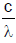)

Solution:

As we know E=hv
and v=c/λ
then E=hc/λ
Now, h and c are constants
So, E is inversely proportional to λ
So, if λ is large then Energy is low or Energy is high then λ is low.
So statement is wrong.
Hence, Option (D) is correct.

QUESTION: 15

Match the column
Column - I and Column - II contain data on Schrondinger Wave - Mechanical model, where symbols have
their usual meanings. Match the columns.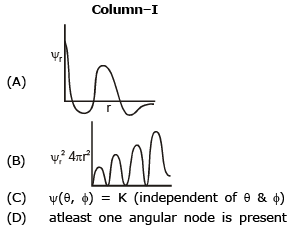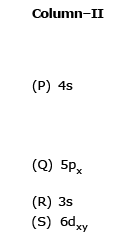Solution: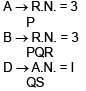QUESTION: 16

Match the column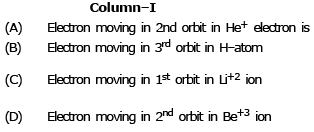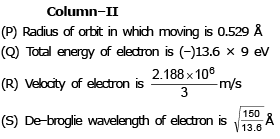Solution: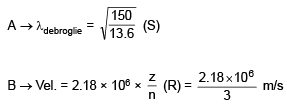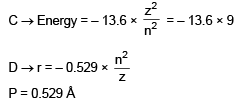QUESTION: 17

The French physicist Louis de-Broglie in 1924 postulated that matter, like radiation, should exhibit a dual behaviour. He proposed the following relationship between the wavelength λ of a material particle, its linear momentum p and planck constant h.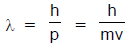The de Broglie relation implies that the wavelength of a particle should decreases as its velocity increases. It also implies that for a given velocity heavier particles should have shorter wavelength than lighter particles. The waves associated with particles in motion are called matter waves or de Broglie waves.
These waves differ from the electromagnetic waves as they,
(i) have lower velocities
(ii) have no electrical and magnetic fields and
(iii) are not emitted by the particle under consideration.
The experimental confirmation of the de–Broglie relation was obtained when Davisson and Germer, in 1927, observed that a beam of electrons is diffracted by a nickel crystal. As diffraction is a characteristic property of waves, hence the beam of electron behaves as a wave, as proposed by de–Broglie.
Werner Heisenberg considered the limits of how precisely we can measure properties of an electron or other microscopic particle like electron. He determined that there is a fundamental limit of how closely we can measure both position and momentum. The more accurately we measure the momentum of a particle, the less accurately we can determine its position. The converse is also ture. This is summed up in what we now call the “Heisenberg uncertainty principle : It is impossible to determine simultaneously and precisely both the momentum and position of a particle. The product of undertainty in the position, Δx and the uncertainity in the momentum Δ(mv) must be greater than or equal to h/4π. i.e.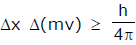Q.

The correct order of wavelength of Hydrogen (1H1), Deuterium (1H2) and Tritium (1H3) moving with same kinetic energy is :

Solution: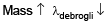QUESTION: 18

The French physicist Louis de-Broglie in 1924 postulated that matter, like radiation, should exhibit a dual behaviour. He proposed the following relationship between the wavelength λ of a material particle, its linear momentum p and planck constant h.The de Broglie relation implies that the wavelength of a particle should decreases as its velocity increases. It also implies that for a given velocity heavier particles should have shorter wavelength than lighter particles. The waves associated with particles in motion are called matter waves or de Broglie waves.
These waves differ from the electromagnetic waves as they,
(i) have lower velocities
(ii) have no electrical and magnetic fields and
(iii) are not emitted by the particle under consideration.
The experimental confirmation of the de–Broglie relation was obtained when Davisson and Germer, in 1927, observed that a beam of electrons is diffracted by a nickel crystal. As diffraction is a characteristic property of waves, hence the beam of electron behaves as a wave, as proposed by de–Broglie.
Werner Heisenberg considered the limits of how precisely we can measure properties of an electron or other microscopic particle like electron. He determined that there is a fundamental limit of how closely we can measure both position and momentum. The more accurately we measure the momentum of a particle, the less accurately we can determine its position. The converse is also ture. This is summed up in what we now call the “Heisenberg uncertainty principle : It is impossible to determine simultaneously and precisely both the momentum and position of a particle. The product of undertainty in the position, Δx and the uncertainity in the momentum Δ(mv) must be greater than or equal to h/4π. i.e.Q.

The transition, so that the de - Broglie wavelength of electron becomes 3 times of its initial value in He+ ion will be :

Solution: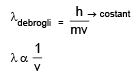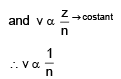i.e. transition from
n = 1 to n = 3, n = 2 to n = 6
n = 3 to n = 9

QUESTION: 19

The French physicist Louis de-Broglie in 1924 postulated that matter, like radiation, should exhibit a dual behaviour. He proposed the following relationship between the wavelength λ of a material particle, its linear momentum p and planck constant h.The de Broglie relation implies that the wavelength of a particle should decreases as its velocity increases. It also implies that for a given velocity heavier particles should have shorter wavelength than lighter particles. The waves associated with particles in motion are called matter waves or de Broglie waves.
These waves differ from the electromagnetic waves as they,
(i) have lower velocities
(ii) have no electrical and magnetic fields and
(iii) are not emitted by the particle under consideration.
The experimental confirmation of the de–Broglie relation was obtained when Davisson and Germer, in 1927, observed that a beam of electrons is diffracted by a nickel crystal. As diffraction is a characteristic property of waves, hence the beam of electron behaves as a wave, as proposed by de–Broglie.
Werner Heisenberg considered the limits of how precisely we can measure properties of an electron or other microscopic particle like electron. He determined that there is a fundamental limit of how closely we can measure both position and momentum. The more accurately we measure the momentum of a particle, the less accurately we can determine its position. The converse is also ture. This is summed up in what we now call the “Heisenberg uncertainty principle : It is impossible to determine simultaneously and precisely both the momentum and position of a particle. The product of undertainty in the position, Δx and the uncertainity in the momentum Δ(mv) must be greater than or equal to h/4π. i.e.Q. If the uncertainty in velocity & position is same, then the uncertainty in momentum will be :

Solution: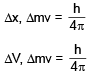multiplying by m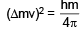QUESTION: 20

The only electron in the hydrogen atom resides under ordinary conditions on the first orbit. When energy is supplied, the electron moves to higher energy orbit depending on the amount of energy absorbed. When this electron returns to any of the lower orbits, it emits energy. Lyman series is formed when the electron returns to the lowest orbit while Balmer series is formed when the electron returns to second orbit. Similarly, Paschen, Brackett and Pfund series are formed when electron returns to the third, fourth and fifth orbits from higher energy orbits respectively. Maximum number of lines produced when an electron jumps from nth level to ground level is equal to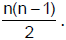. For examle, in the case of n = 4, number of lines produced is 6. (4 → 3, 4 → 2, 4 → 1, 3 → 2, 3 → 1, 2 → 1). When an electron returns from n2 to n1 state, the number of lines in the spectrum will be equal to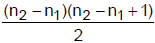If the electron comes back from energy level having energy E2 to energy level having energy E1, then the difference may be expressed in terms of energy of photon as :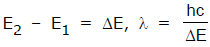Since h and c are constants, ΔE corresponds to definite energy; thus each transition from one energy level to another will produce a light of definite wavelength. This is actually observed as a line in the spectrum of hydrogen atom.
Wave number of line is given by the formula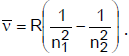where R is a Rydberg’s constant
(R = 1.1 × 107 m-1)

Q.

The energy photon emitted corresponding to transition n = 3 to n = 1 is [h = 6 × 10-34 J - sec]

Solution: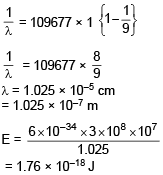QUESTION: 21

The only electron in the hydrogen atom resides under ordinary conditions on the first orbit. When energy is supplied, the electron moves to higher energy orbit depending on the amount of energy absorbed. When this electron returns to any of the lower orbits, it emits energy. Lyman series is formed when the electron returns to the lowest orbit while Balmer series is formed when the electron returns to second orbit. Similarly, Paschen, Brackett and Pfund series are formed when electron returns to the third, fourth and fifth orbits from higher energy orbits respectively. Maximum number of lines produced when an electron jumps from nth level to ground level is equal to. For examle, in the case of n = 4, number of lines produced is 6. (4 → 3, 4 → 2, 4 → 1, 3 → 2, 3 → 1, 2 → 1). When an electron returns from n2 to n1 state, the number of lines in the spectrum will be equal toIf the electron comes back from energy level having energy E2 to energy level having energy E1, then the difference may be expressed in terms of energy of photon as :Since h and c are constants, ΔE corresponds to definite energy; thus each transition from one energy level to another will produce a light of definite wavelength. This is actually observed as a line in the spectrum of hydrogen atom.
Wave number of line is given by the formulawhere R is a Rydberg’s constant
(R = 1.1 × 107 m-1)

Q.

In a collection of H–atom, electrons make transition from 5th excited state to 2nd excited state then maximum number of different types of photons observed are :

Solution: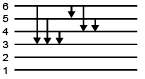QUESTION: 22

The only electron in the hydrogen atom resides under ordinary conditions on the first orbit. When energy is supplied, the electron moves to higher energy orbit depending on the amount of energy absorbed. When this electron returns to any of the lower orbits, it emits energy. Lyman series is formed when the electron returns to the lowest orbit while Balmer series is formed when the electron returns to second orbit. Similarly, Paschen, Brackett and Pfund series are formed when electron returns to the third, fourth and fifth orbits from higher energy orbits respectively. Maximum number of lines produced when an electron jumps from nth level to ground level is equal to. For examle, in the case of n = 4, number of lines produced is 6. (4 → 3, 4 → 2, 4 → 1, 3 → 2, 3 → 1, 2 → 1). When an electron returns from n2 to n1 state, the number of lines in the spectrum will be equal toIf the electron comes back from energy level having energy E2 to energy level having energy E1, then the difference may be expressed in terms of energy of photon as :Since h and c are constants, ΔE corresponds to definite energy; thus each transition from one energy level to another will produce a light of definite wavelength. This is actually observed as a line in the spectrum of hydrogen atom.
Wave number of line is given by the formulawhere R is a Rydberg’s constant
(R = 1.1 × 107 m-1)

Q.

The difference in the wavelength of the 1st line of Lyman series and 2nd line of Balmer series in a hydrogen atom is :

Solution: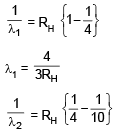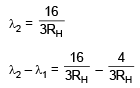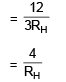QUESTION: 23

The only electron in the hydrogen atom resides under ordinary conditions on the first orbit. When energy is supplied, the electron moves to higher energy orbit depending on the amount of energy absorbed. When this electron returns to any of the lower orbits, it emits energy. Lyman series is formed when the electron returns to the lowest orbit while Balmer series is formed when the electron returns to second orbit. Similarly, Paschen, Brackett and Pfund series are formed when electron returns to the third, fourth and fifth orbits from higher energy orbits respectively. Maximum number of lines produced when an electron jumps from nth level to ground level is equal to. For examle, in the case of n = 4, number of lines produced is 6. (4 → 3, 4 → 2, 4 → 1, 3 → 2, 3 → 1, 2 → 1). When an electron returns from n2 to n1 state, the number of lines in the spectrum will be equal toIf the electron comes back from energy level having energy E2 to energy level having energy E1, then the difference may be expressed in terms of energy of photon as :Since h and c are constants, ΔE corresponds to definite energy; thus each transition from one energy level to another will produce a light of definite wavelength. This is actually observed as a line in the spectrum of hydrogen atom.
Wave number of line is given by the formulawhere R is a Rydberg’s constant
(R = 1.1 × 107 m-1)

Q.

The wave number of electromagnetic radiation emitted during the transition of electron in between two levels of Li2+ ion whose principal quantum numbers sum is 4 and difference is 2 is :

Solution: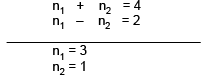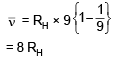QUESTION: 24

A single electron atom has a nuclear charge +Ze where Z is the atomic number and ‘e’ is the electronic charge. It requires 47.2ev to excite the electron from 2nd Bohr’s orbit to 3rd Bohr’s orbit from above given data solve the following question:

Q.

The atomic no. of the element is:

Solution: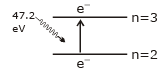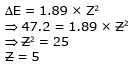QUESTION: 25

A single electron atom has a nuclear charge +Ze where Z is the atomic number and ‘e’ is the electronic charge. It requires 47.2ev to excite the electron from 2nd Bohr’s orbit to 3rd Bohr’s orbit from above given data solve the following question:

Q. The energy required for transition of electron from third to fourth Bohr’s orbit is:

Solution: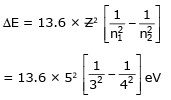= 26.5 ×10–12 erg